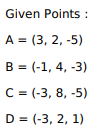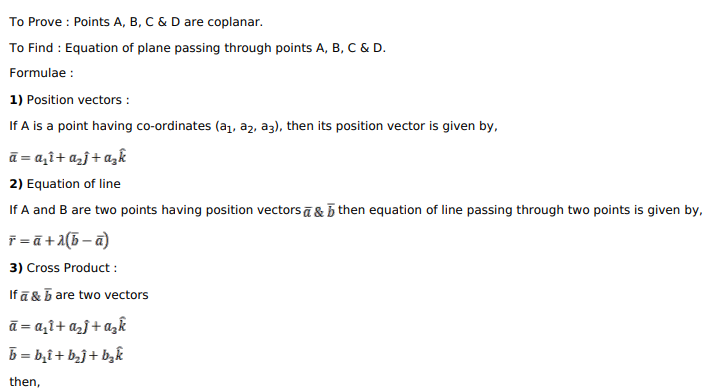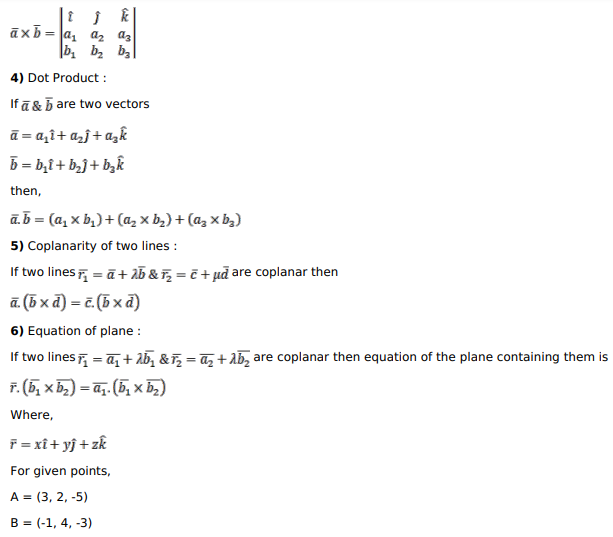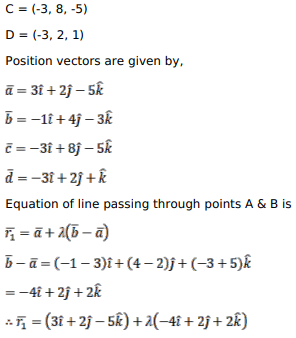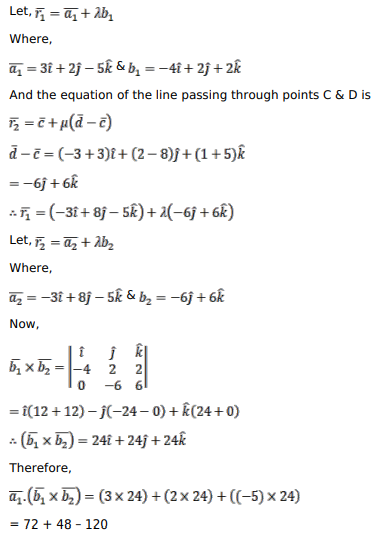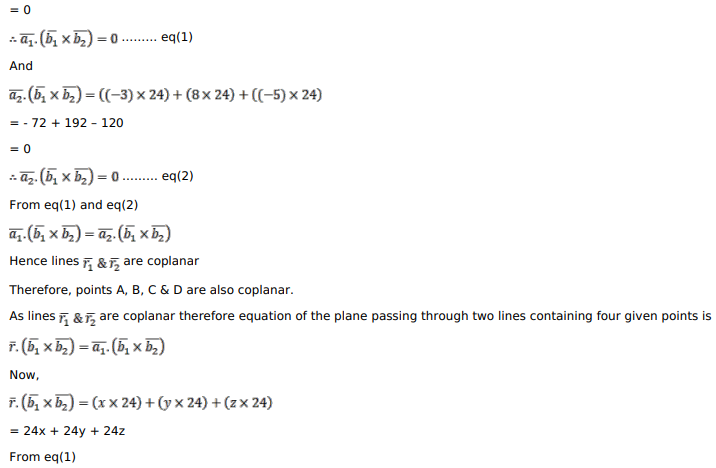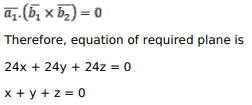# Show that the four pointsQuestion:

Show that the four points $A(3,2,-5), B(-1,4,-3), C(-3,8,-5)$ and $D(-3,2,1)$ are coplanar. Find the equation of the plane containing them.

Solution: